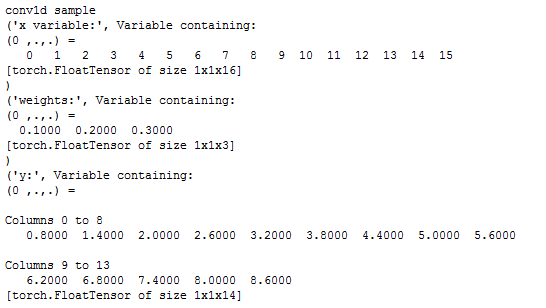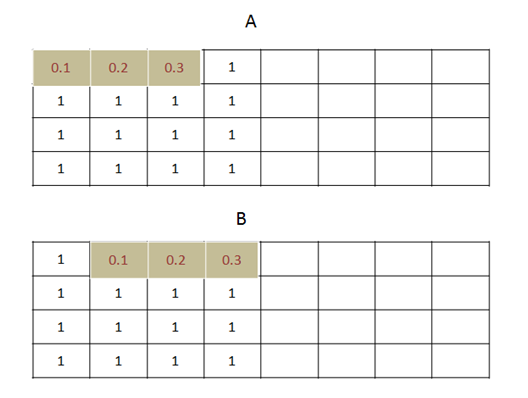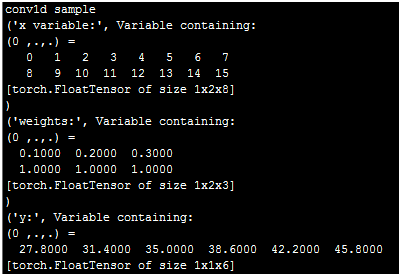conv1d是一维卷积，它和conv2d的区别在于只对宽度进行卷积，对高度不卷积。

torch.nn.functional.conv1d(input, weight, bias=None, stride=1, padding=0, dilation=1, groups=1)

 input：输入的Tensor数据，格式为(batch,channels,W)，三维数组，第一维度是样本数量，第二维度是通道数或者记录数。三维度是宽度。

 weight：卷积核权重，也就是卷积核本身。是一个三维数组，(out_channels, in_channels/groups, kW)。out_channels是卷积核输出层的神经元个数，也就是这层有多少个卷积核；in_channels是输入通道数；kW是卷积核的宽度。

 bias：位移参数，可选项，一般也不用管。

 stride：滑动窗口，默认为1，指每次卷积对原数据滑动1个单元格。

 dilation：卷积核之间的空格，默认1。

 groups：将输入数据分组，通常不用管这个参数，没有太大意义。

import torch

import torch.nn as nn

import torch.nn.functional as F

print("conv1d sample")

a=range(16)

x = Variable(torch.Tensor(a))

x=x.view(1,1,16)

print("x variable:", x)

b=torch.ones(3)

b=0.1

b1=0.2

b2=0.3

weights = Variable(b)#torch.randn(1,1,2,2)) #out_channel*in_channel*H*W

weights=weights.view(1,1,3)

print ("weights:",weights)

print ("y:",y)（1） 原始数据大小是0-15的一共16个数字，卷积核宽度是3，向量是[0.1,0.2,0.3]。 我们看第一个卷积是对x[0:3]共3个值[0,1,2]进行卷积，公式如下：

0*0.1+1*0.2+2*0.3=0.8

（2） 对第二个目标卷积，是x[1:4]共3个值[1,2,3]进行卷积，公式如下：

1*0.1+2*0.2+3*0.3=1.4print("conv1d sample")

a=range(16)

x = Variable(torch.Tensor(a))

x=x.view(1,2,8)

print("x variable:", x)

b=torch.ones(6)

b=0.1

b1=0.2

b2=0.3

weights = Variable(b)

weights=weights.view(1,2,3)

print ("weights:",weights)

print ("y:",y)

[0.1,0.2,0.3]和[1,1,1]

[0,1,2]和[8,9,10]

[1,2,3]和[9,10,11]